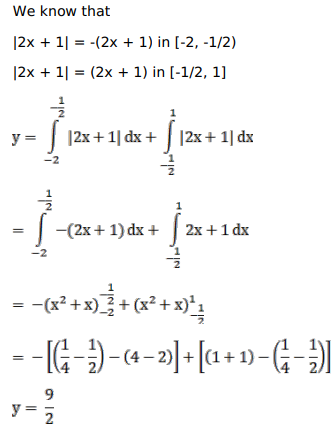# Mark against the correct answer in the following:

Question:

Mark $(\sqrt{ })$ against the correct answer in the following:

$\int_{-2}^{1}|2 x+1| d x=?$

A. $\frac{5}{2}$

B. $\frac{7}{2}$

C. $\frac{9}{2}$

D. 0

Solution: